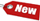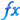Browse Accounting Lessons HereAccounting Terms & DefinitionsAccounting for Merchandising ActivitiesDebits and Credits (Double Entry Accounting)Business Valuation FormulasTime Value of Money & Present/Future ValuesComplex Debt & Equity InstrumentsCommon Stock & Shareholder's EquityAccounting & Finance RatiosValuing Common StockCorporate Income TaxesLower of Cost or Market (LCM) & Inventory ValuationChart of Accounts & BookkeepingBonds Payable & Long Term LiabilitiesCapital AssetsGAAP, Accrual & Cash Accounting, Information Commodity, Internal Controls & MaterialityWhat category of browser are you on this website? Accounting student (homework help) Finance professor (university research) Accounting manager (at work) Other Explore Careers in Accounting and FinanceVisit our section on Careers in Accounting & Finance to explore vast opportunities in this industry.

Common Financial and Accounting Ratios & Formulas

Accounting ratios are among the most popular and widely used tools of financial analysis because if properly analyzed, they help us identify areas that require further analysis on financial statements of corporations. A ratio can help us uncover conditions and trends that are difficult to find by inspecting individual components making up the ratio. For instance, knowing how much cash a company has in the bank might be a little useful, but working out a ratio to determine how much cash a company has, versus how much short term debt it has coming up is a lot more useful. Accounting ratios help us do just that. In fact, accountants admit that interpreting financial data is the most challenging aspect of ratio analysis. First of all, what is a ratio?

A ratio is a mathematical relation between two quantities expressed as a percentage, a rate or proportion. For example a ratio can derive the answer \$900 or can be expressed a 100% or 9:1 or just “9” In this tutorial, we will go over 4 major categories of accounting ratios that are known as the 4 building blocks of financial statement analysis. They are i) liquidity & efficiency ratios ii) solvency ratios iii) profitability ratios and iv)market value ratios.

i) Short Term Solvency or Liquidity RatiosCurrent ratio = Current assets / Current liabilitiesQuick ratio = (Current assets – inventory) / Current liabilitiesCash ratio = Cash / current liabilitiesNet Working Capital = Net working capital / total assetsInternal measure = Current assets / average daily operating costs

ii) Long Term Solvency or Financial Leverage RatiosTotal debt ratio = (Total assets – total equity) / Total assetsDebt to Equity ratio = Total debt / total equityEquity Multiplier = Total assets / total equityLong term debt ratio = Long term debt / (Long term debt + total equity)Times interest earned = Earnings before Interest & Taxes / InterestCash coverage ratio = (Earnings before Interest & Taxes + Depreciation) / Interest

iii) Asset use or turnover ratiosInventory turnover = Cost of goods sold / InventoryDays’ sales in Inventory = 365 days / Inventory turnoverReceivables turnover = Sales / Accounts receivableDays’ sales in receivables = 365 days / Receivables turnoverNet Working Capital (NWC) turnover = Sales / Net Working CapitalFixed asset turnover = Sales / net fixed assetsTotal asset turnover = Sales / total assets

iv) Profitability RatiosProfit margin = Net income / SalesReturn on Assets (ROA) = Net income / total assetsReturn on Equity (ROE) = Net income / Total equityROE = (Net Income / Sales) x (Sales / Assets) x (Assets / Equity)

v) Market Value RatiosPrice to Earnings ratio = Price per share / Earnings per shareMarket-to-book ratio = Market value per share / book value per share